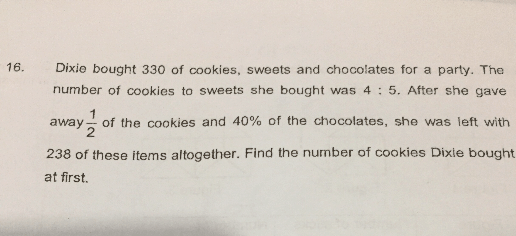# QuestionAppreciate help for this question. Thanks

4 units : 5 units

4 units + 5 units + 100% chocolates = 9 units + 100% chocolates ——-> 330
54 units + 600% ——-> 1980

4 units – (1/2) x 4 units + 5 units + (100% – 40%) chocolates = 7 units + 60% chocolates ——-> 238
70 units + 600% chocolates ——-> 2380

1 units = (2380 – 1980)/(70 – 54) = 25
4 units = 4 x 25 = 100

Ans : 100 cookies.